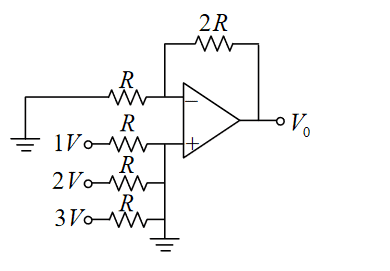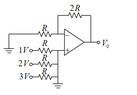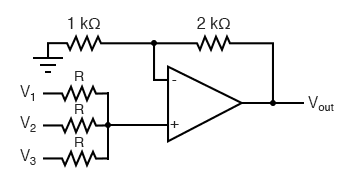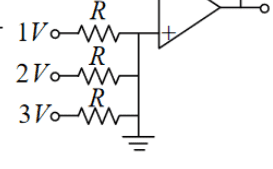# Op-Amp circuit output analysis trouble

• Engineering
• PRITAM the cat of Ne
PRITAM the cat of Ne
Homework Statement
What is the output voltage Vo of the OPAMP circuit given below?
Relevant Equations
V=IRTHE ANSWER IS GIVEN: 6V, but according to me as the positive terminal is grounded the negative terminal will be virtually grounded (0V), from ohm's law (applying to the two series resistance) it is expected that Vo is 0V!

I don't know where I am getting wrong!

Mentor
Welcome to PF.Homework Statement:: What is the output voltage Vo of the OPAMP circuit given below?
Relevant Equations:: V=IR

it is expected that Vo is 0V!
It's kind of a trick question. Since the + input is grounded, it doesn't matter what voltages are on the other sides of the bottom 3 resistors. And 2 x 0 = 0V still.

For it to be a more normal adder, there would have to be a vertical resistor between the summing junction at the + input and the ground connection. That would form a weighted voltage divider, no? Or you could just unground the + input, which would give you the average voltage of the inputs:#### Attachments

boo
The noninverting terminal is NOT at zero volts. Do a KVL there and you will find that the voltage there (and thus at the inverting terminal as well) is equal to 2*V. Then do a KVL at the inverting terminal and you will find that the gain is, indeed, 6.

Mentor
The noninverting terminal is NOT at zero volts. Do a KVL there and you will find that the voltage there (and thus at the inverting terminal as well) is equal to 2*V. Then do a KVL at the inverting terminal and you will find that the gain is, indeed, 6.
Welcome to PF.

Which circuit are you referring to? The schematic in the OP (original post, post #1) has the + input for the opamp hard-tied to ground, no? If it's a hard tie to ground, it doesn't matter what else you connect to that node...

boo
Of course you're right.

•berkeman
boo
OK, so here's why I still think that the non-inverting terminal has voltage = 2*V and not zero. I assume that this problem assumes IDEAL voltage sources. Now by placing that ground at the end of those 3 parallel voltages sources (hanging off the non-inverting terminal) you have effectively shorted them out. You have made the voltage on the "positive" end of that circuit equal to the "negative" end with both at ground potential.

Now the problem here is that you cannot short out an ideal voltage source. By definition an ideal voltage source will deliver ANY current to maintain the stipulated voltage. Thus KCL at the non-inverting terminal will still show 2*V volts (and thus the output voltage 6*V).

Now, in practice 1) no one would wire a circuit like this (unless they were trying to start a fire) and 2) if these voltage sources were wired like this in practice the "batteries" would be current limited and unable to sustain that voltage. They would, indeed, short out and the voltage would drop to zero.

So, I think that what this circuit was supposed to demonstrate is the limitations of the "ideal" voltage source model that our calculations are supposed to be based upon. If you like I can easily post an example of the calculation of a thevenin equivalent circuit which gives the WRONG answer for the thevenin resistance if a parallel voltage source is removed after it is short circuited. Let me know if you would like me to do this.

Last edited by a moderator:
Mentor
OK, so here's why I still think that the non-inverting terminal has voltage = 2*V and not zero. I assume that this problem assumes IDEAL voltage sources. Now by placing that ground at the end of those 3 parallel voltages sources (hanging off the non-inverting terminal) you have effectively shorted them out. You have made the voltage on the "positive" end of that circuit equal to the "negative" end with both at ground potential.

Now the problem here is that you cannot short out an ideal voltage source. By definition an ideal voltage source will deliver ANY current to maintain the stipulated voltage. Thus KCL at the non-inverting terminal will still show 2*V volts (and thus the output voltage 6*V).
No, there is nothing strange going on here, except for the circuit schematic error (or connection error if somebody tried to build this). No power supplies are stressed, no assumptions of ideal opamps, etc. The problem is just that the error keeps those 3 voltage sources from being able to change the voltage of the + opamp input. The current in each of those 3 branches is just ##\frac{V_i}{R}##, and those 3 currents are sinked to ground leaving 0V at the + input.Gold Member
The noninverting terminal is NOT at zero volts. Do a KVL there and you will find that the voltage there (and thus at the inverting terminal as well) is equal to 2*V. Then do a KVL at the inverting terminal and you will find that the gain is, indeed, 6.
The non-inverting terminal is at zero volts. The inverting terminal is therefore at zero volts, unless you are given an offset voltage or you assume the opamp will run against the rails and you are given that voltage or some additional information you have not posted. The KVL equations do not give a gain of 6. You have:
$$V_+ = 0$$
$$\frac {(0-V_{-})} {R} + \frac {(V_{out}-V_{-})} {2R} = 0$$
If the opamp is ideal, then ##V_{-} = 0V##. In that case, no current flows through R and no current flows through 2R, i.e., ##V_{out}=0V##. The only way to get a non-zero answer is if you are given an offset voltage or some non-zero bias current. Personally, I think the figure given is a mistake if the answer of 6V is supposed to be correct. If I were to go measure some random opamp using only the information given in the configuration shown, are telling me that I'll get ##6V## as an output and that I can choose any value at all for R? I'm willing to wager that won't be the case.

•berkeman